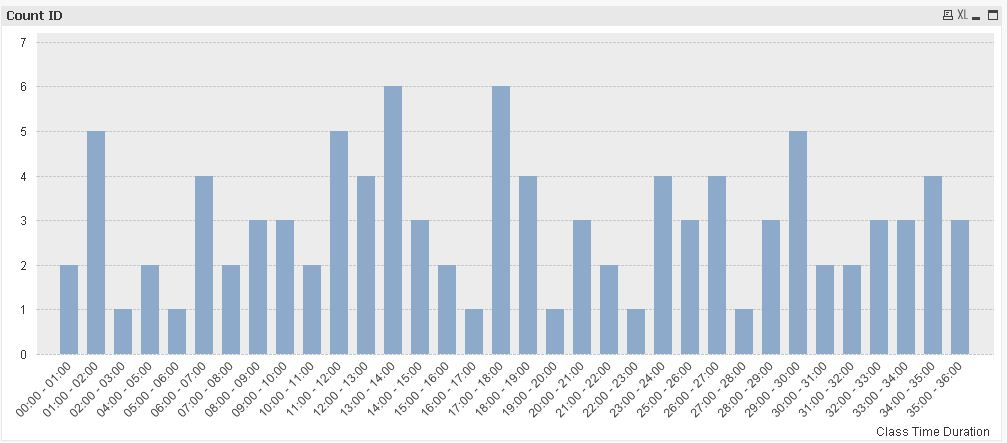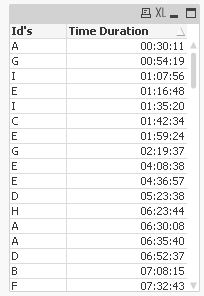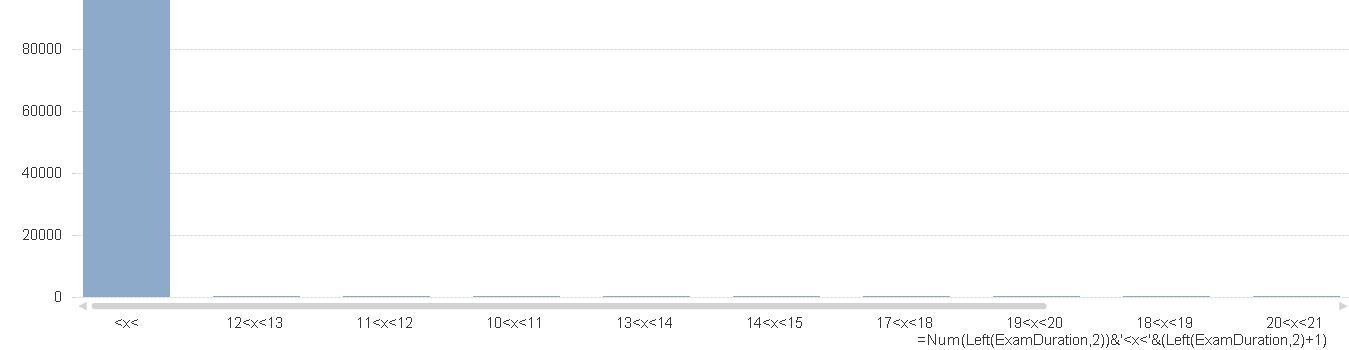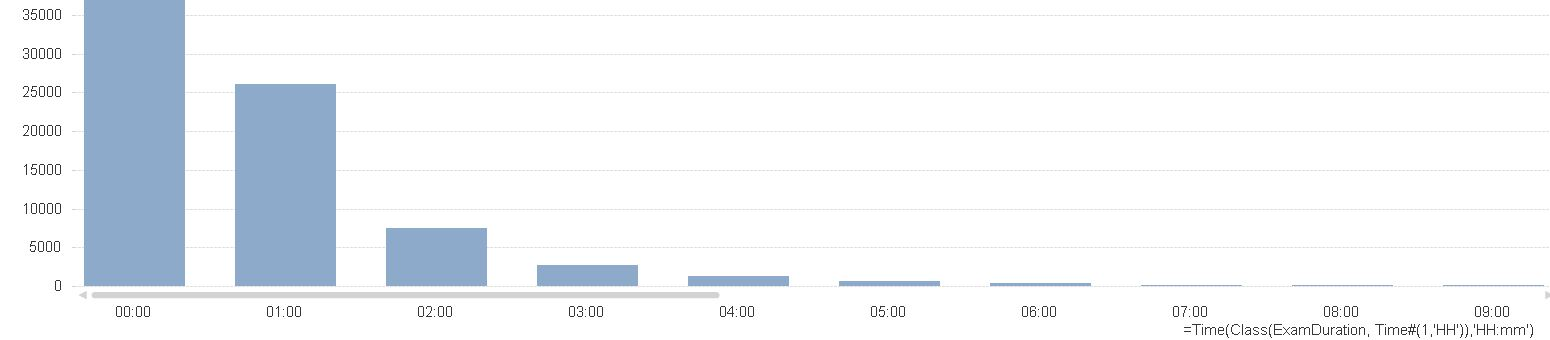# QlikView App Development

Discussion Board for collaboration related to QlikView App Development.

Announcements
Coming your way, the Qlik Data Revolution Virtual Summit. October 27-29. REGISTER
cancel
Showing results for
Did you mean:
HighlightedNot applicable

## Calculated dimension of time ranges on x-axis

I have a table like shown below

Time DurationId's
00:45A
00:35A
01:10B
01:16B

I wanted to plot a graph with Time Duration on X-axis and Count(Id's) on Y-axis (as expression). But on X-axis i wanted time duration as time range, i.e. 0 < x < 1 and 1 < x < 2 (count of id's with time duration between 0 and 1 hour; and 1 and 2 hours).

I used class function to group time duration like, Class([Time Duration], Time#(1,'HH')). It worked, but the problem is with the visualization. The X-axis scale is showing like 0 < x < 0.0034 and 0.004 < x < 0.0063 (some decimal number, i just wanted to indicate the how it is being show). How to make Class function understand that it is time that i am trying to display.

1 Solution

Accepted Solutions
HighlightedMVP

with some more Buckets:hope this helps

regards

Marco

13 Replies
HighlightedNot applicable

Hi,

You can use Calculated Dimension in chart.

=if(Left(TIME,2)>=0 and Left(TIME,2)<1,'0<X<1',
if(Left(TIME,2)>=1 and Left(TIME,2)<2,'1<X<2',
if(Left(TIME,2)>=2 and Left(TIME,2)<3,'2<X<3')))

You need to write Conditional Statement for 0-23 hrs( I have shown just 3)HighlightedNot applicable

Hi Kuntal

Thank you for your quick response. This is a good idea. This works in the limits are known (for example, 24 hours in a day). But this field being duration/interval it can go beyond 24 hours. How can i handle this case.

HighlightedMVP

Hi

You can use something like this for the dimension:

=Interval(Class([Time Duration], 1/24), 'HH')

or

=(Class([Time Duration], 1/24)) * 24

The class() function returns a dual with a value equal to the upper or lower limit of the range.

HTH

Jonathan

Logic will get you from a to b. Imagination will take you everywhere. - A Einstein
HighlightedNot applicable

You can use following Expression

=Num(Left(TIME,2))&'<x<'&(Left(TIME,2)+1)

I think it will solve thepurpose.

HighlightedNot applicable

Hi Jonathan

Thanks for your response. Is it possible to show it like '00:00 < x < 01:00'; '01:00 < x < 02:00'; so on.... This clearly explains to the viewer that the values are for Time Duration between 0 and 1 hour and so on. Just showing bars at  00:00; 01:00;02:00 and so on...is not making the intention very obvious.

HighlightedNot applicable

Hi Kuntal

I tried your suggestion and it is closer to what i want but not there yet. Here is how it looked.HighlightedNot applicable

Can u pls send me a rough snapshot of data.

as I am getting the correct output.HighlightedNot applicable

That data is too big, for a rough snapshot it looks something like the table i had in my first message. With the same data when i tried Jonathan's suggestion it looked liked belowFirst bar says values between 00:00 and 00:01 hours and so on....

HighlightedMVP

Hi,you might use

=Dual(

Interval(SubField(Class([Time Duration], 1/24),'<= x <',1),'hh:mm')&' - '&

Interval(SubField(Class([Time Duration], 1/24),'<= x <',2),'hh:mm'),

Class([Time Duration], 1/24)

)

as calculated dimension.

hope this helps

regards

Marco